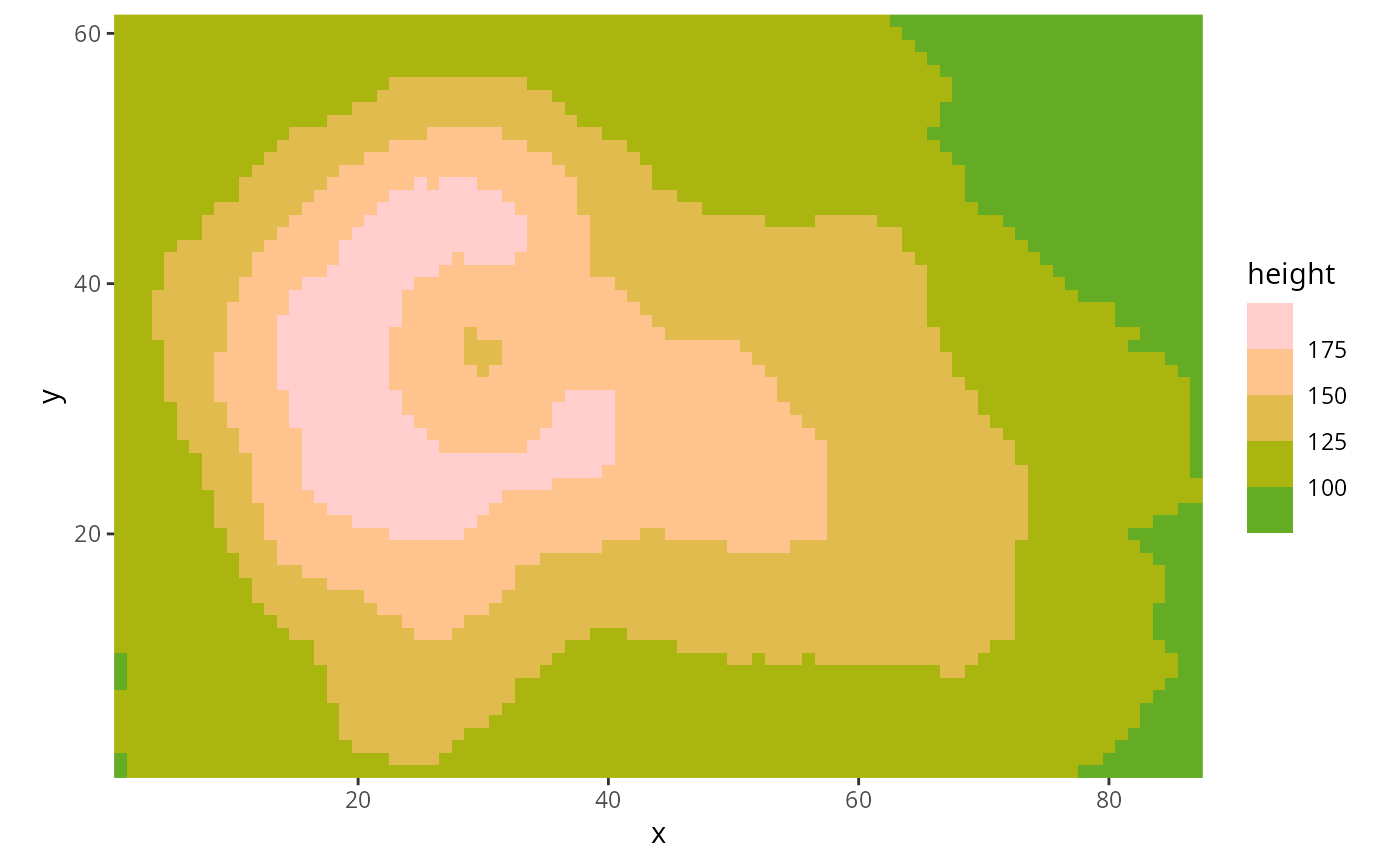Binned ggplot2 color scales using the color palettes generated by sequential_hcl.

scale_colour_binned_sequential(
palette = NULL,
c1 = NULL,
c2 = NULL,
cmax = NULL,
l1 = NULL,
l2 = NULL,
h1 = NULL,
h2 = NULL,
p1 = NULL,
p2 = NULL,
alpha = 1,
rev = TRUE,
begin = 0,
end = 1,
na.value = "grey50",
guide = "coloursteps",
aesthetics = "colour",
n_interp = 11,
...
)

scale_color_binned_sequential(
palette = NULL,
c1 = NULL,
c2 = NULL,
cmax = NULL,
l1 = NULL,
l2 = NULL,
h1 = NULL,
h2 = NULL,
p1 = NULL,
p2 = NULL,
alpha = 1,
rev = TRUE,
begin = 0,
end = 1,
na.value = "grey50",
guide = "coloursteps",
aesthetics = "colour",
n_interp = 11,
...
)

scale_fill_binned_sequential(..., aesthetics = "fill")

## Arguments

palette The name of the palette to be used. Run hcl_palettes(type = "sequential") for available options. Beginning chroma value. Ending chroma value. Maximum chroma value. Beginning luminance value. Ending luminance value. Beginning hue value. Ending hue value. If set to NA, generates a single-hue scale. Control parameter determining how chroma should vary (1 = linear, 2 = quadratic, etc.). Control parameter determining how luminance should vary (1 = linear, 2 = quadratic, etc.). Numeric vector of values in the range [0, 1] for alpha transparency channel (0 means transparent and 1 means opaque). If TRUE (default), reverses the order of the colors in the color scale (compared to sequential_hcl). Number in the range of [0, 1] indicating to which point in the color scale the smallest data value should be mapped. Number in the range of [0, 1] indicating to which point in the color scale the largest data value should be mapped. Color to be used for missing data points. Type of legend. Use "coloursteps" for color bar with discrete steps. The ggplot2 aesthetics to which this scale should be applied. Number of discrete colors that should be used to interpolate the binned color scale. 11 will work fine in most cases. common binned scale parameters: name, breaks, labels, and limits. See binned_scale for more details.

## Details

If both a valid palette name and palette parameters are provided then the provided palette parameters overwrite the parameters in the named palette. This enables easy customization of named palettes.

Compared to sequential_hcl the ordering of the colors in the sequential ggplot2 scale are reversed by default (i.e., rev = TRUE) to be more consistent with ggplot2's own scales such as scale_color_fermenter. For most named palettes this leads to darker and more colorful colors for larger values on the scale. This is typically the better default on light/white backgrounds.

## Examples

library("ggplot2")

# volcano plot
df <- data.frame(height = c(volcano), x = c(row(volcano)), y = c(col(volcano)))
ggplot(df, aes(x, y, fill = height)) +
geom_raster() + scale_fill_binned_sequential(palette = "Terrain", rev = FALSE) +
coord_fixed(expand = FALSE)